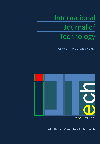### Recognizing Offline Handwritten Mathematical Expressions (ME) based on a Predictive Approach of Segmentation using K-NN Classification

Sachin Naik, Pravin Metkewar

Corresponding email: sachin.naik@sicsr.ac.in

Published at : 29 Jul 2015
IJtech : IJtech Vol 6, No 3 (2015)
DOI : https://doi.org/10.14716/ijtech.v6i3.1069

Naik, S., & Metkewar, P. 2018. Recognizing Offline Handwritten Mathematical Expressions (ME) based on a Predictive Approach of Segmentation using K-NN Classification. International Journal of Technology. Volume 6(3), pp.345-354

302
 Sachin Naik Symbiosis Institute of Computer Studies and Research(SICSR), A Constituent of Symbiosis International University (SIU), Atur Centre, Model Colony, PUNE-411016, Maharashtra State, India Pravin Metkewar Symbiosis Institute of Computer Studies and Research(SICSR), A Constituent of Symbiosis International University (SIU), Atur Centre, Model Colony, PUNE-411016, Maharashtra State, India
Email to Corresponding Author

AbstractRecognition of handwritten mathematical expressions has been an important topic for many researchers for decades. It remains one of the most challenging and exciting areas in pattern recognition. In the recognition process of offline handwritten mathematical expressions, segmentation is the most important process. Problems in ambiguities of identifying superscript and subscript in complex offline mathematical expressions remain one of the most important problem. To the best of our knowledge little work has been done in the segmentation of offline handwritten mathematical expressions with respect to superscript and subscript. In this paper an efficient segmentation technique for superscript, subscript and main characters within offline handwritten mathematical expressions has been proposed. This technique is based on the generation of predictions for superscript, subscript and main characters within handwritten mathematical expressions, which helps for the reconstruction of mathematical expressions during the recognition process with their spatial interrelationship. The proposed system was conducted as an experiment with a database of 300 samples of scanned mathematical expressions that comprised 2,000 symbols out of which there were 31 different types of Mathematical Symbols. The classification of the elements was carried out by the K-NN-classifier based on density features. This experiment shows remarkable results.

Features extraction, K-NN classification, Mathematical Expressions (ME) Recognition, Segmentation

Anderson, R.H., 1968. Syntax-directed Recognition of Hand-printed Two-dimensional Mathematics. Ph.D. Dissertation, Dept. Eng. Appl. Phys., Harvard Univ., Cambridge, MA

Chan, K-C., Yeung, D-Y., 2000. Mathematical Expression Recognition: A Survey. IJDAR 3: pp. 3–15, Springer-Verlag

Davila, K., Ludi, S., Zanibbi, R., 2014. Using Off-line Features and Synthetic Data for On-line Handwritten Math Symbol Recognition. Proceeding The 14th International Conference on Frontiers in Handwriting Recognition (ICFHR)

Escalera, Sergio, Fornés, Alicia, Sánchez, Gemma, Lladós, Joseph, 2009. Blurred Shape Model for Binary and Grey-level Symbol Recognition. Pattern Recognition Letters, Volume 30(15), pp. 1424-1433

Fateman, R., Tokuyasu, T., Berman, B., Mitchell, N., 1996. Optical Character Recognition and Parsing of Typeset Mathematics. Journal of Visual Communication and Image Representation, Volume 7(1), pp. 2-15

Garain, U., Chaudhuri, B.B., 2005. Segmentation of Touching Symbols for OCR of Printed Mathematical Expressions: An Approach based on Multifactorial Analysis. Proceedings of the Eight International Conference on Document Analysis and Recognition (ICDAR’05), IEEE.

Ha, T.M., Zimmermann, M., Bunke, H., 1998. Off-line Handwritten Numeral String Recognition by Combining Segmentation-based and Segmentation-free Methods. Pattern Recognition, Volume 31(3), pp. 257-272

Hu, Y., Peng, L., Tang, Y., 2014. Online-handwritten Mathematical Expression Recognition Method based on Statistical and Semantic Analysis, Document Analysis System (DAS), The 11th IAPR International Workshop, pp. 171-175, IEEE

Kanahori, T., Tabata, K., Cong, W., Tamari, F., Suzuki, M., 2000. On-line Recognition of Mathematical Expressions using Automatic Rewriting Method, ICMI, LNCS 1948, Springer, pp. 394-401

Kenichi, T., Naoya, Y., Kenji, M., Takayuki, K., Kensaku, M., Yasuhito, S., Tomoichi, T., 2004. A Study of Symbol Segmentation Method for Handwritten Mathematical Formula Recognition using Mathematical Structure Information. Proceedings of the 17th International Conference on Pattern Recognition (ICPR, 2004), pp. 630-633

Lee, H.J., Wang, J.S., 1995. Design of Mathematical Expression System. In: Proceedings of ICDAR’95, Canada, pp. 1084-1087

Lu, C., Mohan, K., 2013. Recognition of Online Handwritten Mathematical Expressions, Project Final Report, Stanford University

Matan, Burges, J.C., 1991. Recognizing Overlapping Hand-printed Characters by Centered-objects Integrated Segmentation and Recognition. In: Proceedings of International Joint Conference on Neural Networks (IJCNN), pp. 504-511, Seattle, USA

Plamondon, R.J., Sargur, N.S., 2000. On-line and Off-line Handwriting Recognition: A Comprehensive Survey. IEEE Transactions on Pattern Analysis and Machine Intelligence, Volume 22(1), pp. 63-84

Salim, O., Mouldi, B., Abderrazak, L., 2007. Segmentation and Recognition of Handwritten Numeric Chains. Journal of Computer Science, Volume 3(4), pp. 242-248

Simistira, Papavssiliou, Katsouros, Carayannis, 2014. Recognition of Spatial Relations in Mathematical Formulas. Proceeding The 14th International Conference on Frontiers in Handwriting Recognition (ICFHR),pp. 164-168, IEEE

Stefan, L., Winkler, H.J., Lang, M., 1996. A Soft-decision Approach for Symbol Segmentation within Handwritten Mathematical Expressions. In: Proceeding International Conference on Acoustics, Speech, and Signal Processing (ICASSP), pp. 3434-2437

Tapia, E., Rojas, R., 2007. A Survey on Recognition of Online Handwritten Mathematical Notation Freie University at Berlin. Institute fur Informatik,Germany

Zanibbi, R., Blostein, D., 2012. Recognition and Retrieval of Mathematical Expressions. IJDAR 2012, Springer-Verlag

Zanibbi, R., Blostein, D., James, R.C., 2002. Recognizing Mathematical Expressions using Tree Transformation. IEEE Transactions on Pattern Analysis and Machine Intelligence, Volume 24(11), pp. 1455-1467